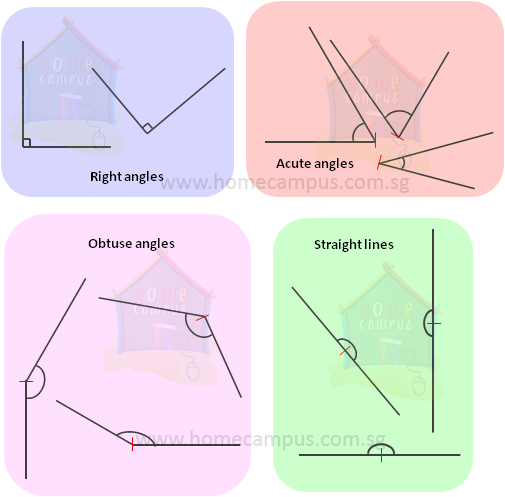What is an Angle and how to measure it?

Practice Unlimited Questions

1. What is an angle?

An angle is the amount of curve between two lines meeting or crossing at a point.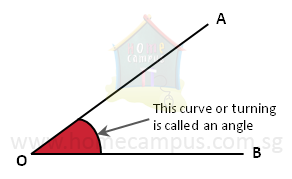is used to denote an angle.

In the figure above, lines AO and OB meet at O to make the angle AOB or AOB.
O is the vertex of AOB.

2. How do we measure angles?

Angles are measured in degrees using a protractor.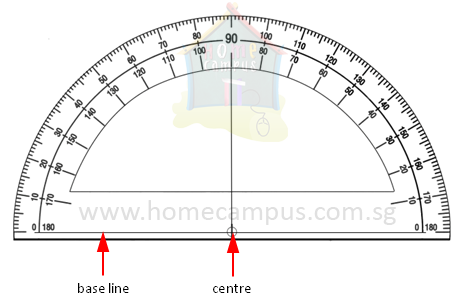To measure the angle EFG between two lines EF and FG drawn below: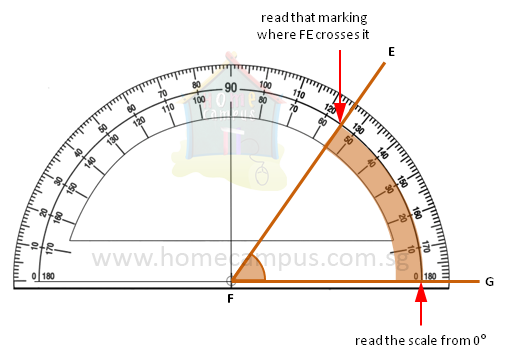1. Place the base line of the protractor over one of the lines, say, FG.
2. Place the centre of the protractor on the vertex i.e. F.
3. Read the scale starting from 0° at FG to where FE crosses it. That reading is EFG.

In our case, EFG = 55°

Another example: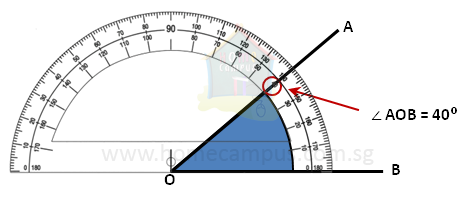3. Special angles

Following are some special angles:

1. Right angle
When two lines form an angle of 90°, the angle is known as a right angle and the two lines are said to be perpendicular to each other.

2. Acute angle
Angles less than 90° are acute angles.

3. Obtuse angle
Angles more than 90° are obtuse angles.

4. Straight line
When two lines meet at 180° they make a straight line.